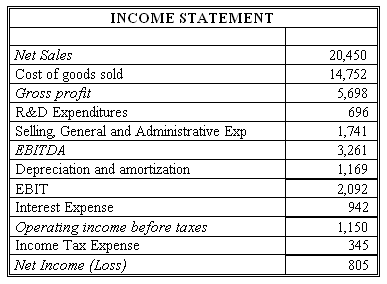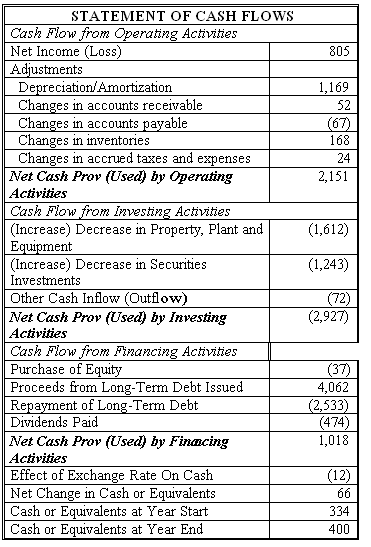### CFA Practice Question

There are 290 practice questions for this topic.

### CFA Practice Question

Connie's Sporting Goods (CSG) has net income of \$805 million for 2011. Using information from the CSG's financial statements below, use the EBIT approach to find what FCFF and FCFE should be for CSG. Assume the income tax rate is 30%.Correct Answer: FCFF = EBIT (1 - Tax rate) + Dep - FCInv - WCInv
= 2092 (1 - 0.3) + 1169 - 1612 - (-52 + 67 - 168 - 24) = \$1,198 million

FCFE = EBIT (1 - Tax rate) - Int (1 - Tax rate) + Dep - FCInv - WCInv + Net borrowing = 2092 (1 - 0.3) - 942 (1 - 0.3) + 1169 - 1612 - (-52 + 67 - 168 - 24) + (4062 - 2533) = \$2,068 million

User Comment
blackberry1 CAN SOMEONE EXPLAIN NET WORKING CAPITAL PART? LIKE WHY IS -52 AND NOT POSITIVE? THNKS
aravinda You can think of it this way....

Any increases in current liability (ex: AP) is increase in cashflow and any decrease in current asset is a decrease in cashflow...
In the calculation of CFO, these 'signs' are already taken into consideration...so for the actual values you just the opposite sign...

hope I was clear...
Allen88 Thanks aravinda!
quanttrader yes, since CFO = NI + NCC - WC(inv), then as aravinda has said we will use opposite signs since FCFF = CFO + int(1-t) - FC(inv)
davidt876 lackberry1 - you can just use the numbers as they appear. i agree that flipping all their signs just to multiply their sum by (-1) seems a little redundant

but you should understand why an increase in current assets - like inventory and receivables - represent a cash outflow.. in the same way an increase in fixed (tangible) assets represents a cash outflow and is subtracted as well